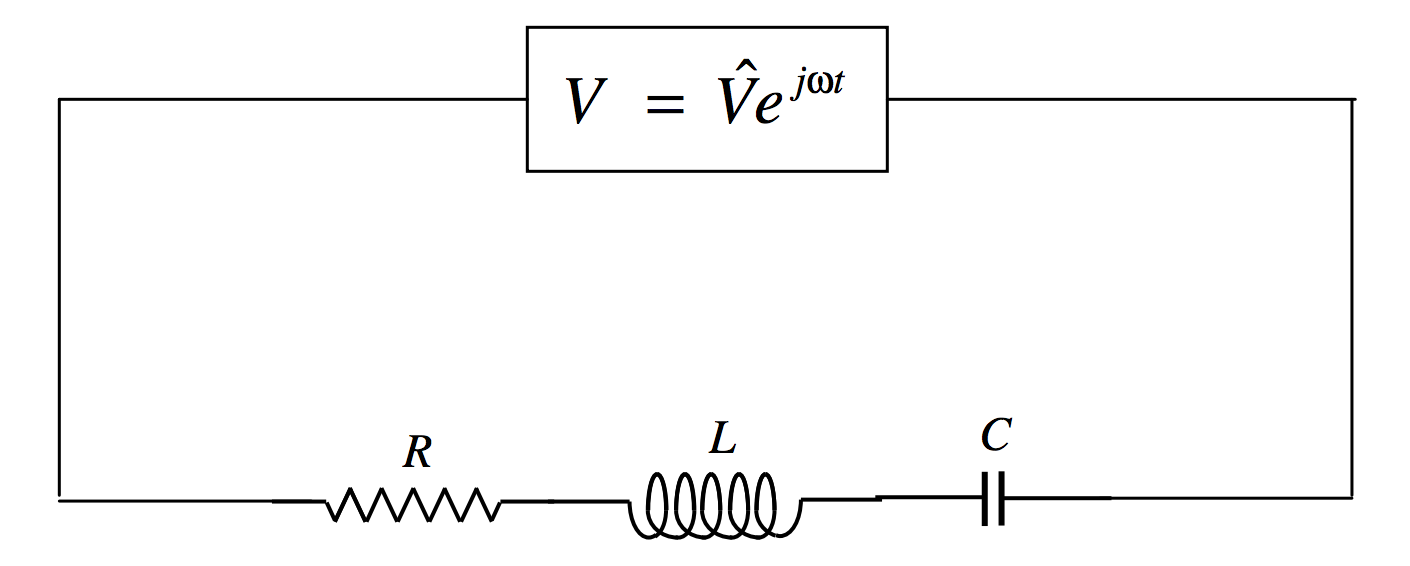$$\require{cancel}$$

# 13.7: The RLC Series Acceptor Circuit

•• Contributed by Jeremy Tatum
• Emeritus Professor (Physics & Astronomy) at University of Victoria

A resistance, inductance and a capacitance in series is called an "acceptor" circuit, presumably because, for some combination of the parameters, the magnitude of the inductance is a minimum, and so current is accepted most readily. We see in Figure $$\text{XIII.5}$$ an alternating voltage $$V=\hat{V}e^{j\omega t}$$ applied across such an $$R$$, $$L$$ and $$C$$.FIGURE $$\text{XIII.5}$$

The impedance is

$\label{13.7.1}Z=R+j\left (L\omega -\dfrac{1}{C\omega}\right ).$

We can see that the voltage leads on the current if the reactance is positive; that is, if the inductive reactance is greater than the capacitive reactance; that is, if $$\omega > 1/\sqrt{LC}$$. (Recall that the frequency, $$\nu$$, is $$\omega/(2\pi)$$). If $$\omega < 1/\sqrt{LC}$$, the voltage lags behind the current. And if $$\omega = 1/\sqrt{LC}$$, the circuit is purely resistive, and voltage and current are in phase.

The magnitude of the impedance (which is equal to $$\hat{V}/\hat{I}$$) is

$\label{13.7.2}|Z|=\sqrt{R^2+\left (L\omega - 1/(C\omega )\right )^2},$

and this is least (and hence the current is greatest) when $$\omega = 1/\sqrt{LC}$$, the resonant frequency, which I shall denote by $$\omega_0$$.

It is of interest to draw a graph of how the magnitude of the impedance varies with frequency for various values of the circuit parameters. I can reduce the number of parameters by defining the dimensionless quantities

$\label{13.7.3}\Omega = \omega / \omega_0$

$\label{13.7.4}Q=\dfrac{1}{R}\sqrt{\dfrac{L}{C}}$

and

$\label{13.7.5}z=\dfrac{|Z|}{R}.$

You should verify that $$Q$$ is indeed dimensionless. We shall see that the sharpness of the resonance depends on $$Q$$, which is known as the quality factor (hence the symbol $$Q$$). In terms of the dimensionless parameters, Equation \ref{13.7.2} becomes

$\label{13.7.6}z=\sqrt{1+Q^2(\Omega -1/\Omega )^2}.$

This is shown in Figure $$\text{XIII.6}$$, in which it can be seen that the higher the quality factor, the sharper the resonance.FIGURE $$\text{XIII.6}$$

In particular, it is easy to show that the frequencies at which the impedance is twice its minimum value are given by the positive solutions of

$\label{13.7.7}\Omega^4 -\left (2+\dfrac{3}{Q^2}\right )\Omega^2+1=0.$

If I denote the smaller and larger of these solutions by $$\Omega_- \text{ and }\Omega_+$$, then $$\Omega_+ -\Omega_-$$ will serve as a useful description of the width of the resonance, and this is shown as a function of quality factor in Figure $$\text{XIII.7}$$.FIGURE $$\text{XIII.7}$$